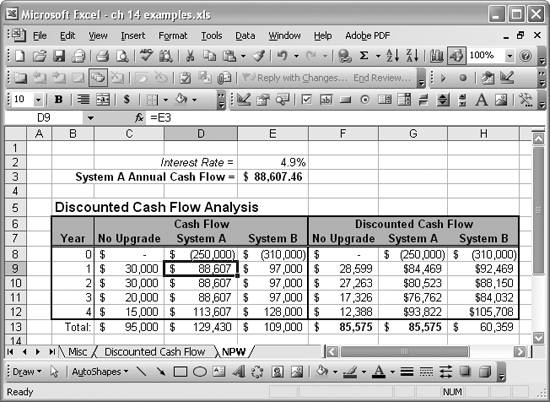# Solving Inverse Problems

Problem

You want to solve a problem in reverse. For example, referring to the net present worth example discussed in Recipe 14.8, you might want to find what annual cash flow value would make one upgrade option the more attractive alternative.

Solution

Use Goal Seek or Solver to iteratively find a solution. See Chapters 9 and 13 for an in-depth discussion and examples of using Goal Seek and Solver.

Discussion

Let's reconsider the example discussed in Recipe 14.8. Earlier it was found that the No Upgrade option yielded the highest net present worth. Now the question is what annual cash flow would be required for, say, the system A option to yield a net present worth equal to or greater than the No Upgrade option. You can solve this problem with just a few small changes to the spreadsheet shown earlier in Figure 14-2. All you have to do is add a new value in a cell that represents the assumed annual cash flow for system A. Then you need to revise the cash flow values shown in the undiscounted cash flow column for system A to refer to the new assumed value. Finally, you can use Goal Seek to iteratively find the assumed cash flow value while setting the net present worth value for system A to that of the No Upgrade option. Figure 14-3 shows the result of this exercise.

Cell E3 contains the new assumed annual cash flow value for system A. After running Goal Seek as discussed a moment ago, the resulting value is \$88,607, and you can see that the net present worth values for the No Upgrade and System A options in cells F13 and G13, respectively, are the same. Therefore, in order for system A to be the more attractive option, your annual cash flow for that option must exceed \$88,607.

The only changes I made to the original spreadsheet to find this solution are as follows:

1. I added a value in cell E3 to hold the assumed annual cash flow value for system A.
2. I added a text label in cell D3.
3. I changed cells D9, D10, and D11 from hardcoded values to the cell formula =E3.
4. I changed the formula in cell D12 from =85000+0.1*ABS(D8) to =E3+0.1*ABS(D8).

After making these changes, I ran Goal Seek to set cell G13 to a value of 85,575 by changing the value in cell E3. The result is as shown in Figure 14-3.

Figure 14-3. Net present worth example revisitedBe sure to read Chapter 9 to learn a lot more about using Solver and Goal Seek.Excel Scientific and Engineering Cookbook (Cookbooks (OReilly))
ISBN: 0596008791
EAN: 2147483647
Year: N/A
Pages: 206
Authors: David M Bourg